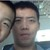# 青岛版数学九年级下册第五章第四节二次函数的图象和性质课件

• 10人评价）
• 95人阅读
• 上传者：王玉冬

### 相关文档推荐2积分 下载
```5.4二次函数的图象
和性质（3）

抛物线           开口方向          对称轴        顶点坐标

直线X=0
开口向下                     (0,0)
y  0.5x2                     或y轴
直线X=0
开口向下                     (0,1)
y   0.5x2  1                  或y轴
直线X=0
开口向下                      (0,-1)

y  0.5x2  1                  或y轴
2
y=ax                                        (0,0)顶点在原点
a﹥0   开口向上      直线X=0
y=ax2+k          a﹤0   开口向下      或y轴        (0,k)顶点在y轴

填表:
抛物线       开口方向      对称轴     顶点坐标

开口向上     直线X=0     (0, 0)
y  2x 2
开口向上     直线X=1     (1, 0)
y  2(x 1)2
开口向上     直线X=-1    (-1, 0)
y  2(x 1)2

y=a(x-h)2  a﹥0   开口向上 直线X=h   (h, 0)
a﹤0   开口向下
顶点在x轴上

K>0 上加
y=ax2           y=ax2+k
K<0 下减

左加
y=ax2           y=a(x-h)2
右减

1 会用描点法画出二次函数
y  a(x  h)2  k

2 经历探索和发现二次函数
y  a(x  h)2  k

3经历观察，推理和交流等过程，获得研究

1
y      (x  1)2  1
2
的开口方向、顶点与对称轴、

(2)描点时,一般先定顶点,然后根据对称性,描出对称点
(3)连线时,注意顶点附近的大致走向,画出的抛物线应

(4)对描点,连线中出现的误差,要适当修正,或修正不合

解:  先列表
x    …   -4 -3  -2 -1  0  1   2  …

1     …         -1.5              …
y   (x 1)2 1 -5.5 -3 -1 -1.5 -3 -5.5
2
再描点、连线             直线x=－1
y
1
(1)抛物线       1
y   (x 1)2 1
2           -5 -4 -3 -2 -1 o1 2 3 4 5 x

-2
-3
抛物线        1
y    (x 1)2 1        -4
2
的开口向下,                         -5
-6
对称轴是直线x=－1,                    -7
-8
-9
顶点是(－1, －1).                  -10
1
y   (x 1)2 1
2
1     2
观察二次函数          1 2    1 2   y   (x 1) 1
y   x , y   x 1, 2
在同一直角坐标系中的图象，思考这三条抛物线2 2
有什么关系？
形状相同，
开口方向相同.

顶点不同，

1      对称轴不同
y   x2 ,       .
1                      2
y   (x 1)2 1
2

1
y   x2 1,
2

抛物线    1   怎样移动就可以得到抛物线              1   2？
y   x2                    y   (x 1) 1
2                             2
y
1
-5   -4  -3  -2  -1     o1     2   3   4   5       x
-1
-2
1
平移方法1:                                                  y          (x  1)2     1
2                     -3
向下平移                                                                   -4

1   2                                     1                                       -5
y       x                            y          x2   1
2           1个单位                          2                                        -6
向左平移                                                                               -7
1                                            -8
y              (x    1)2       1
1个单位                               2                                            -9
平移方法2:                                                                                 -10x=－1
向左平移                                           向下平移
1                                        1                                                    1                 2
y       x2                          y           (x  1)2                            y              (x   1)         1
2           1个单位                         2                  1个单位                              2
归纳小结

一般地，抛相同物线y                                            a(    x       h  )22       k与       y        ax      形状            _____，
位不同   置      ____。把抛物线y                                            ax2向上（下）向左（右）
平移，可以得到抛物线y                                                             a()    x      h2           k。
平移的h、方k                  向、距离要根据_____的值来决定。

抛物线向上y                     a(向下x        h)2          k有如下特点：
（1）当a                       0时，开口____；当a                                                   0，开口___；
（2）对称轴是直线x=h                                         ____；
（3）顶点坐标是（h，k）                                  ______。
二次函数y=a(x-h)2+k的图象和性质

１.顶点坐标与对称轴
２.位置与开口方向
３.增减性与最值

抛物线       y=a(x-h)2+k(a>0)       y=a(x-h)2+k(a<0)
顶点坐标         （h，k）                  （h，k）
对称轴         直线x=h                  直线x=h

位置       由h和k的符号确定              由h和k的符号确定
开口方向           向上                    向下

在对称轴的左侧,y随着x的增大而减小.  在对称轴的左侧,y随着x的增大而增大.
增减性     在对称轴的右侧, y随着x的增大而增大. 在对称轴的右侧, y随着x的增大而减小.
最值      当x=h时,最小值为k.          当x=h时,最大值为k.
一般地,抛物线y=a(x－h)2＋k

y=ax2向上(下)向右(左)平移,可以得到

平移方法:
向左(右)平移
y=ax2         y=a(x－h)2 向上(下)平y=a(x－h)2+k
|h|个单位          移|k|个单位

向左(右)平
y=ax2 向上(下)平   y=ax2+k          y=a(x－h)2+k
移|k|个单位          移|h|个单位
各种形式的二次函数的关系

y = a( x - h )2  + k
左                   上
右                   下
平                   平
移                   移
y = ax2 + k           y = a(x - h )2

上下平移            左右平移
y = ax2

结论: 一般地，抛物线         y = a(x-h)2+k
与y = ax2形状相同，位置不同。
1.完成下列表格:
二次函数        开口方向     对称轴     顶点坐标

y=2(x+3)2+5     向上   直线x=－3    (－3,5)
y=－3(x－1)2－2     向下     直线x=1  (1,－2)

y = 4(x－3)2＋7    向上    直线x=3    (3,7)

y=－5(2－x)2－6     向下    直线x=2    (2,－6)
2.请回答抛物线y = 4(x－3)2＋7由抛物线y=4x2怎
样平移得到?
3.抛物线y =－4(x－3)2＋7能够由抛物线y=-4x2平移
得到吗?
3、如何平移：

3                                            3               2
y      (x  1)2                           y          (x   1)          2
4                                            4

3                                                3
y          (x      3)2       3                y          (x      5)2       2
4                                                4
4、抛物线y=a(x+2)2-8经过点（0，0），则a=       2 。

5、抛物线y=3x2向右平移3个单位再向下平移2个

6、抛物线y=2(x+m)2+n的顶点是（-m,n）。
1、填表

向上          （0,3）         y轴
向下           （1,0）       x=1
向上          （-5,2）       x=-5
向上            低
2、抛物线     y  2(x  3) 2  的开口5      ，有最          点，

向下              x=3
3、抛物线     y  3(x  3) 2 的开口2     ，对称轴是           ，

4、把抛物线           向左平移1个单位，然后向上平移3个单位，则
y  x 2
2

2积分下载